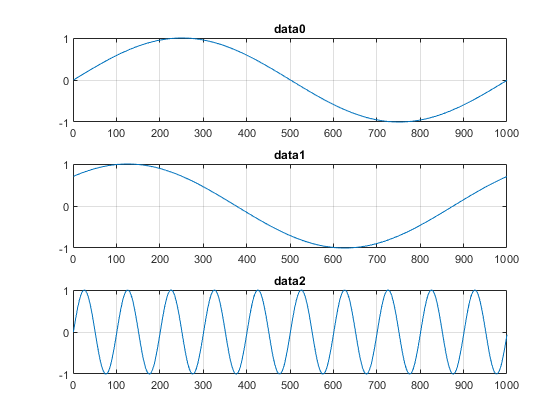# Generate Continuous and Background Signals Using NI Devices

This example shows how to generate analog output data using non-blocking commands. This allows you to continue working in the MATLAB command window during the generation. This is called background generation. Use foreground generation to cause MATLAB to wait for the entire data generation to complete before you can execute your next command.

### Create a DataAcquisition and Add Analog Output Channels

Use `daq` to create a DataAcquisition. This example uses an NI 9263 module in National Instruments® CompactDAQ Chassis NI cDAQ-9178. This is module 2 in the chassis. Output data on three channels at a rate of 10000 scans per second.

```dq = daq("ni"); dq.Rate = 10000; addoutput(dq, "cDAQ1Mod2", 0:2, "Voltage"); ```

### Create Synchronized Signals

Generate output signals by creating a pattern of data that is repeatedly written to the output device. The data for each channel is column based and the output signals are synchronized to a common clock.

Create 3 waveforms:

• `data0`: 1 cycle of a sine wave

• `data1`: 1 cycle of a sine wave with a 45 degree phase lag

• `data2`: 10 cycles of a sine wave

```data0 = sin(linspace(0, 2*pi, 1001))'; data1 = sin(linspace(0, 2*pi, 1001) + pi/4)'; data2 = sin(linspace(0, 2*pi*10, 1001))'; ```

The above waveform contains sin(0) and sin(2*pi). To repeat the waveform coherently, omit the final point.

```data0(end) = []; data1(end) = []; data2(end) = []; ```

At a generation rate of 10000 scans per second, you can expect to observe `data0` and `data1` as 10 Hz sine waves and `data2` as a 100 Hz sine wave.

```subplot(3,1,1) plot(data0) title('data0') grid on subplot(3,1,2) plot(data1) title('data1') grid on; subplot(3,1,3) plot(data2) title('data2') grid on; ```### Queue the Output Data and Start Background Generation

Before starting a continuous generation, `preload` half a second of data. Use `start` to initiate the generation and return control to the command line immediately, allowing you to do other operations in MATLAB while the generation is running in the background.

```preload(dq,repmat([data0, data1, data2], 5, 1)); start(dq, "repeatoutput") ```

Use `pause` in a loop to monitor the number of scans output by the hardware for the duration of the generation.

```t = tic; while toc(t) < 1.0 pause(0.1) fprintf("While loop: scans output by hardware = %d\n", dq.NumScansOutputByHardware) end fprintf("Generation has terminated with %d scans output by hardware\n", dq.NumScansAcquired); ```
```While loop: scans output by hardware = 1109 While loop: scans output by hardware = 2089 While loop: scans output by hardware = 3100 While loop: scans output by hardware = 4095 While loop: scans output by hardware = 5093 While loop: scans output by hardware = 6094 While loop: scans output by hardware = 7082 While loop: scans output by hardware = 8082 While loop: scans output by hardware = 9088 While loop: scans output by hardware = 10099 Generation has terminated with 0 scans output by hardware ```

### Stop the Continuous Background Generation

Background generation runs simultaneously with other operations in MATLAB. Explicitly call `stop` to end the background generation.

```stop(dq) ```

### Generate Output Data Dynamically Using MATLAB Functions

To dynamically generate the output data using a MATLAB function, assign the function to the `ScansRequiredFcn` of the DataAcquisition. The code below is functionally equivalent to `'repeatoutput'`

`dq.ScanRequiredFunction = (src,evt) write(src, repmat([data0, data1, data2], 5, 1));`

`start(dq, "continuous")`

## SupportGet trial now